Open in App
Not now

# Reflection of Light at Plane Surface

• Last Updated : 26 Sep, 2022

Reflection of Light at Plane Surfaces occurs whenever a ray of light strikes or falls over a smooth or polished surface and gets strikes back. In simple words, the ray of light approaching or striking any surface results in the reflection of the light. the ray of light which approaches the reflecting surface or incident over it is known as an incident ray and the ray which reflects back after the strike is known as a reflected ray. If a perpendicular is to be drawn between the incident and reflected rays on the reflecting surface, it is known as a Normal. Let’s Learn more about the Reflection of Light on Plane Surfaces and the Reflection on a Plane Mirror in this article!

## What is Reflection of Light?

The bouncing back of light from the reflected surface is known as the reflection of light. there are certain laws that a ray obeys through which reflection of light occurs.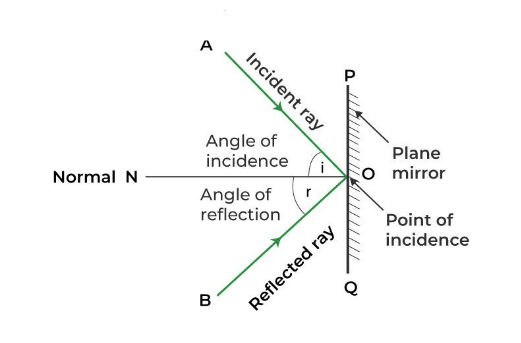There are two main Laws of Reflection:

1. The angle of incidence (∠ i) is equal to the angle of reflection(∠ r) Mathematically, (∠ i = ∠ r).
2. All three rays i.e. the incident ray reflected ray, and the normal at the point of incidence, all lie in the same plane.

## Reflection of Light at Plane Surfaces

### When a Real Object is Infront of the Mirror

Consider a real object such as a candle in front of a plane mirror, now observe the image formed, the image is erect, the same size, and off-course virtual in nature and the distance between the candle and the image formed of that candle from the mirror both are equal, so we can say that if the object is real than the image formed will be the virtual, erect and same size as of object.

### When an Object moves toward or gets away the Plane Mirror

Now suppose that a person starts moving a candle toward the mirror, then the image of the candle approaches toward mirror at the same speed, same goes for when the candle moves away from the mirror, the speed of the image relative to the object will be given by,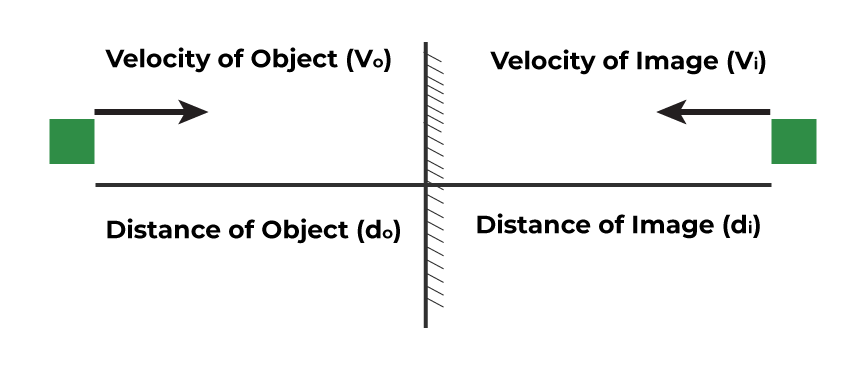Velocity of object = vo

velocity of image = vi  = -vo

The relative speed of image with respect to object = vo – vi = vo – (-vo) = 2 vo .

### When the Mirror moves toward or gets away from the Object

If a person moves a mirror toward or away from the object with the speed v, then the image will appear moving toward or away from the object with the speed 2v.

### Object making certain Angle or Deviation

Through the law of reflection we know that angle of reflection is equal to the angle of reflection, Deviation (∅) is defined as the angle between the direction of the incidence ray and the emergent ray, Suppose that if the ray of the light incident over the surface with angle i, then the deviation is given as: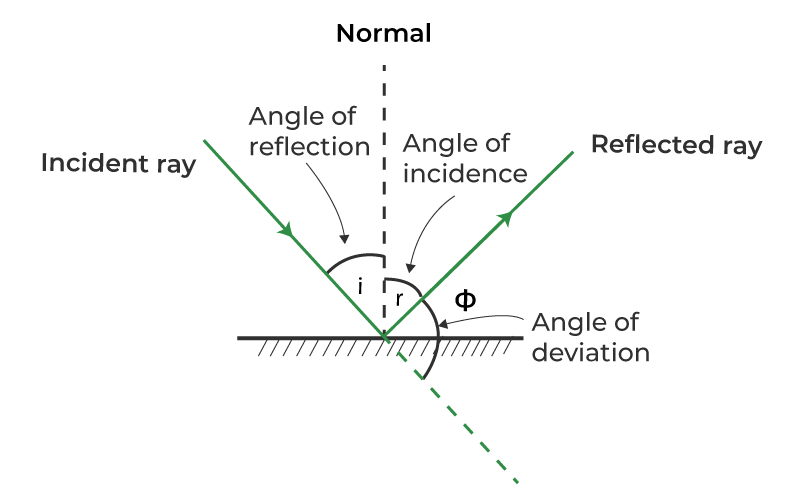Angle of deviation, ∅ = 180 – ( i + r) = 180 – 2i ,

the maximum deviation is occur when angle of incidence is 0°,

max = 180 – 2 × 0 = 180°

### Mirror is Rotated through a certain Angle

Consider the object placed at a fixed position from the mirror, if a plane mirror is rotated through an angle θ, then the reflected ray also rotates through an angle of twice of a rotating plane mirror, i.e. (2θ). But the size of the image remains unchanged.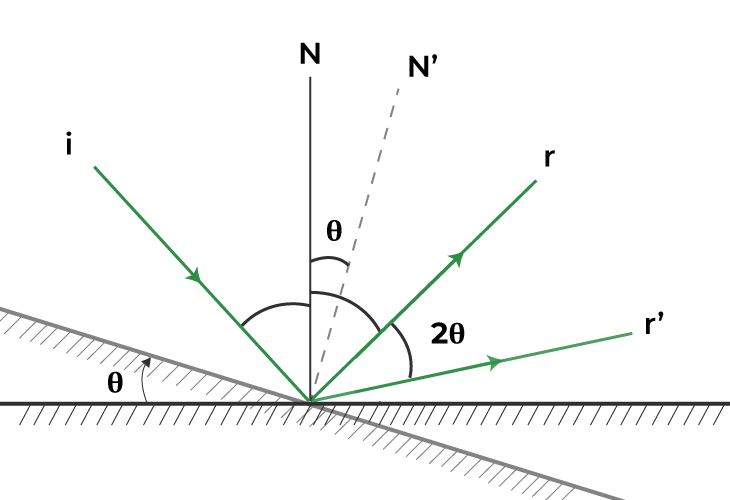## Reflection on a Plane Mirror

When the light rays which gets strokes over the flat mirror and get reflected back. According to the laws of reflection, the angle of incidence is equal to the angle of reflection (∠ i = ∠ r). The image which is present in the mirror is obtained behind the plane. the image is virtual and erect in nature is known as a reflection on a plane mirror.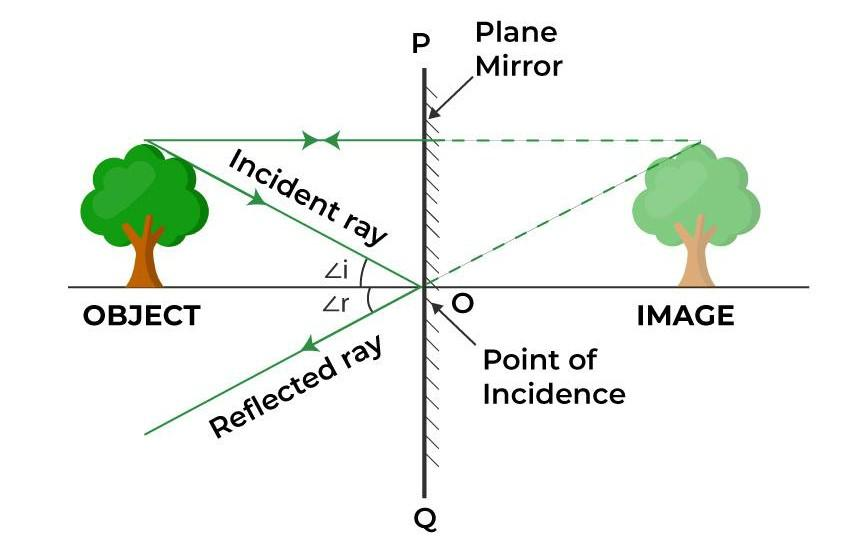### Characteristics of Image formed by Plane Mirror

There are different characteristics formed by the plane mirror these are:

1. The image formed through the plane mirror is always virtual (the image that cannot be obtained on-screen) and erect (Upright or straight).
2. The size of the object and the size of the image both are equal.
3. The distance at which the object is placed is the same as the distance between the image obtained.
4. Laterally inverted images i.e. the right side of the object appears on the left side behind the mirror, are obtained.

## FAQs on Reflection of Light at Plane Surfaces

Question 1: Mention some characteristics of the image formed by the plane mirror.

Some of the characteristics of the image formed by a plane mirror are as:

• Image is always virtual and erect.
• The size of the object and the size of the image both are equal.
• The distance at which the object is placed is the same as the distance between the image obtained.
• The image formed is laterally inverted.

Question 2: If the object is at 30 cm from the plane mirror then determine how far is image form from it.

As we know, the size of the object and the size of the image both are equal in-plane mirrors.

Therefore we have,

The object would be at a distance = 30 + 30 cm = 60 cm from its image.

Question 3: If the angle of incidence formed by a ray is 60° in-plane mirror then what should be the angle of reflection?

The angle of incidence = 60°

For plane mirror,

The angle of incidence (∠ i  ) = angle of reflection (∠ r ).

Therefore Angle of reflection = 60°.

Question 4: Define the term Lateral inversion.

Lateral inversion is defined as the reversal of mirror image where the right side of the object appears on the left side behind the mirror.

For example, when a person lifts his left hand in front of the mirror it appears to be right w.r.t to the mirror.

Another example is the word AMBULANCE written over hospital’s ambulance which is inverted written this is because when a driver saw the word in the mirror it inverts back which makes the word readable so that a person give side to the ambulance.

Question 5: When a plane mirror is rotated through angle θ, what will be the effect on the size of the image and angle of reflection?

When a mirror is rotated through angle θ, then the angle of reflection becomes 2θ, but there is no effect on the size of the image, the in-plane mirror size of the image is equal to the object.

Question 6: Write some properties of Real images.

Some properties of the Real image are:

1. Real Images are Inverted
2. Real Images are formed on the screen.
3. Real images are formed by the concave mirror.
4. Due to the actual intersection of light rays real images are formed.

## Related Articles

My Personal Notes arrow_drop_up
Related Articles